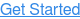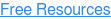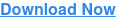# Our top CAS tricks for VCE Maths Methods students

Given our extensive experience figuring out exactly how to use win back time during an exam, we've defined some super strategies for using CAS calculators. Our SuperClasses weekly tutoring students would know how life-changing these tips and our bonus notes are, but we thought we'd make our best advice accessible to everyone. This article includes our top three for VCE Maths Methods students.

These tips are exerpts from our VCE Maths Methods CAS handbooks for TI-Nspire and Casio ClassPad. These downloadable guides are incredibly detailed resources that will help you make the most of your CAS calculator in many commonly examined scenarios. Access them free here.

## 1. Differentiation: defining derivative equations

Questions requiring differentiation appear multiple times in the calculator exam, so by mastering the following tips and commands, you can buy yourself a lot of precious time in the exam.

Hopefully by now you can see how useful it is to define functions – the derivative function is no different.

For example, in the screen on the right, we defined g(x) as being the derivate of f(x). We can do this by using the differentiation icon found in your keyboard under Math2, then defining the expression by highlighting it all then going to Interactive, Define.Here’s a piece of advice: instead of choosing another letter or number to define the derivative by, just chuck a d in front of whatever function you’re differentiating. That way, you’re not going to get confused about what derivative belongs to which function.

For example, in the following screen, we defined df1(x) as being the derivate of f1(x) using menu, 1: Actions, 1: Define and menu, 4: Calculus, 1: Derivative. Now we can simply use in whatever calculations or substitutions we need.## 2. Antidifferentiation: using integrals to calculate area

There are two types of areas you can be asked to calculate – signed and absolute – and the method for doing each is different.

The signed area involves taking the area bounded by a graph and the x-axis, regardless of whether that area is negative or positive. On the other hand, when finding the absolute area, you do have to be aware of any ‘negative’ area and make it positive.

For example, let’s say we had the graph of y = x (x - 2) ( x - 4) and we were interested in the area bounded by the graph between x = 0 and x = 4.

If we were calculating the signed area, we wouldn’t bother checking whether the area was above or below the x-axis – we would just integrate between x = 0 and x = 4.However, if we were after the absolute area, we’d need to graph the equation first and calculate area above the x-axis and area below the x-axis separately. As you can see from the graph below, the area between x = 2 and x = 4 is negative. We can make it positive by either:

• Multiplying the integral by -1 (as multiplying two negatives creates a positive)
• Swapping the terminals

The moral to take away from the above is that the signed area does not represent the true area bounded by the graph and the x-axis – what happens is that the negative area actually subtracts from the positive area, resulting in a lower value than expected. In contrast, the absolute area gives you the actual area (as it involves making any negative area positive), so it is the one that crops up in the exam more often.

For example, let’s say we had the graph of y = x (x - 2) ( x - 4) and we were interested in the area bounded by the graph between x = 0 and x = 4.

If we were calculating the signed area, we wouldn’t bother checking whether the area was above or below the x-axis – we would just integrate between x = 0 and x = 4.However, if we were after the absolute area, we’d need to graph the equation first and calculate area above the x-axis and area below the x-axis separately. As you can see from the graph below, the area between x = 2 and x = 4 is negative. We can make it positive by either:

• Multiplying the integral by -1 (as multiplying two negatives creates a positive)
• Swapping the terminals

The moral to take away from the above is that the signed area does not represent the true area bounded by the graph and the x-axis – what happens is that the negative area actually subtracts from the positive area, resulting in a lower value than expected. In contrast, the absolute area gives you the actual area (as it involves making any negative area positive), so it is the one that crops up in the exam more often.

## 3. Probability: binomial distributions

Probability is one of students’ most despised/feared topics. That’s why by making it your strength, you can lap up more marks in the exam that other students would avoid or struggle with.

When you have a situation where there are only two outcomes (typically either success or fail), but the attempts can be repeated any number of times without changing the probability of those outcomes, then the situation can be described by a binomial distribution. Below, we’ll look at some common questions that get asked in relation to binomial distributions and how to calculate them.

When you head to Interactive, Distributions/Inv. Dist, Discrete, you’ll notice that there are two types of binomial commands – Pdf and Cdf. These abbreviations stand for Probability Distribution Function and Cumulative Distribution Function, respectively. From the names, we can logically infer that the Pdf command gives us the probability of obtaining one particular outcome (e.g. 4 successes out of 6 attempts), whereas the Cdf command will accumulate or add probabilities together (e.g. 4 or more successes out of 6 attempts). It’s crucial you pick the right one.

Example (VCAA 2008, Exam 2) [GA exam]

Sharelle is a goal shooter for her netball team. During her matches, she has many attempts at scoring a goal. Assume that each attempt at scoring a goal is independent of any other attempt. In the long term, her scoring rate has been shown to be 80% (that is, 8 out 10 attempts to score a goal are successful).

ii. What is the probability, correct to four decimal places, that exactly 6 of her first 8 attempts at scoring a goal in a match are successful?

iii. What is the probability, correct to three decimal places, that her first 4 attempts at scoring a goal are successful, given that exactly 6 of her first 8 attempts at scoring a goal in a match are successful?

First, in probability questions you must identify the type of distribution. Here there are many repeated attempts, and the outcomes are only success/fail, so this in a binomial distribution.

For Part (ii), we’re asked for exactly 6 of 8 goals to be successful – the word ‘exactly’ here is a big hint that we need to use binomialPdf. When filling out the pop-up box for Binomial Pdf, make sure you input the probability as a decimal. The ‘X Value’ refers to the number of successes you want.Part (iii) is challenging. The word ‘given’ is a dead giveaway that this question involves conditional probability. When you’re dealing with conditional probability, always identify the outcome you already know has happened, and the outcome you’re finding the probability of. In this case, we know that Sharelle has scored 6/8 goals, so that’s what’s given (it goes on the bottom of our fraction). What we want to know is the probability that the first four in a row are successful. Bear in mind that we still need Sharelle to score 6 goals overall, so we need the first four in a row to be successful, and then any 2 of the next 4 to be successful. The way we’d calculate this is as follows:When you head to menu, 5: Probability, 5: Distributions, you’ll notice that there are two types of binomial commands – pdf and cdf. These abbreviations stand for Probability Distribution Function and Cumulative Distribution Function, respectively. From the names, we can logically infer that the pdf command gives us the probability of obtaining one particular outcome (e.g. 4 successes out of 6 attempts), whereas the cdf command will accumulate or add probabilities together (e.g. 4 or more successes out of 6 attempts). It’s crucial you pick the right one.

Example (VCAA 2008, Exam 2) [GA exam]

Sharelle is a goal shooter for her netball team. During her matches, she has many attempts at scoring a goal. Assume that each attempt at scoring a goal is independent of any other attempt. In the long term, her scoring rate has been shown to be 80% (that is, 8 out 10 attempts to score a goal are successful).

ii. What is the probability, correct to four decimal places, that exactly 6 of her first 8 attempts at scoring a goal in a match are successful?

iii. What is the probability, correct to three decimal places, that her first 4 attempts at scoring a goal are successful, given that exactly 6 of her first 8 attempts at scoring a goal in a match are successful?

First, in probability questions you must identify the type of distribution. Here there are many repeated attempts, and the outcomes are only success/fail, so this in a binomial distribution.

For Part (ii), we’re asked for exactly 6 of 8 goals to be successful – the word ‘exactly’ here is a big hint that we need to use D: Binomial Pdf. When filling out the pop-up box for Binomial Pdf, make sure you input the probability as a decimal. The ‘X Value’ refers to the number of successes you want.Part (iii) is challenging. The word ‘given’ is a dead giveaway that this question involves conditional probability. When you’re dealing with conditional probability, always identify the outcome you already know has happened, and the outcome you’re finding the probability of. In this case, we know that Sharelle has scored 6/8 goals, so that’s what’s given (it goes on the bottom of our fraction). What we want to know is the probability that the first four in a row are successful. Bear in mind that we still need Sharelle to score 6 goals overall, so we need the first four in a row to be successful, and then any 2 of the next 4 to be successful. The way we’d calculate this is as follows:## What next?

When push comes to shove, using your CAS calculator is a skill. And just like with any other skill, getting good at it requires lots of patient practice. Now that you've started your journey of CAS discovery, the best way to increase efficiency and accuracy is to work through past calculator-allowed VCAA exams (denoted as 'Exam 2' on the VCAA website). As you clock up your exam question mileage, you'll start to notice certain CAS techniques that are assessed again and again, and others that you seem to have trouble with and need to practice more before exam day rolls around.

Happy calculating!## The Connect CAS Calculator Handbook.

Whether you use a Casio ClassPad or a TI-Nspire, this handbook is your ultimate guide to becoming a CAS guru.